# JS对象分类

2020-10-16

1.构造函数就是可以构造出对象的函数
2.封装:把细节写到一个函数里，别人只需要调用函数并且传个参数(宽度)
3.函数也是对象
4.js之父为了让new可以运行提前规定:

prototype属性自带constructor。
constructor的值等于函数本身。
5.共有属性与原型的关系：包含与被包含。

### 对象需要分类吗？

正方形

let square={
width:5,
getArea(){
return this.width * this.width
},
getLength(){
return this.width * 4
}
}


let squareList=[]
for(let i=0;i<12;i++){
squareList={
width:5,
getArea(){
return this.width * this.width
},
getLength(){
return this.width * 4
}
}
}


width不全是5怎么办？

let squareList=[]
let widthList=[5,6,7,8,5,6,7,5,5,4,5,6]
for(let i=0;i<12;i++){
squareList[i]={
width:widthList[i],
getArea(){
return this.width * this.width
},
getLength(){
return this.width * 4
}
}
}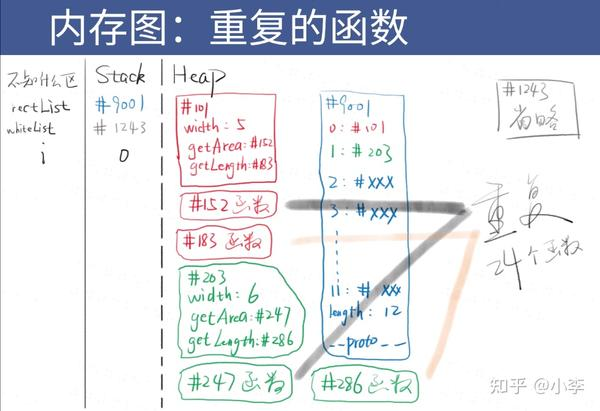借助原型，将12个对象的共用属性放到原型里

let squareList=[]
let widthList=[5,6,7,8,9,5,6,7,8,9,5,6]
let squarePrototype={
getArea(){
return this.width * this.width
},
getLength(){
return this.width * 4
}
}
for(let i=0;i<12;i++){
squareList[i]=Object.create(squarePrototype)
squareList[i].width= widthList[i]
}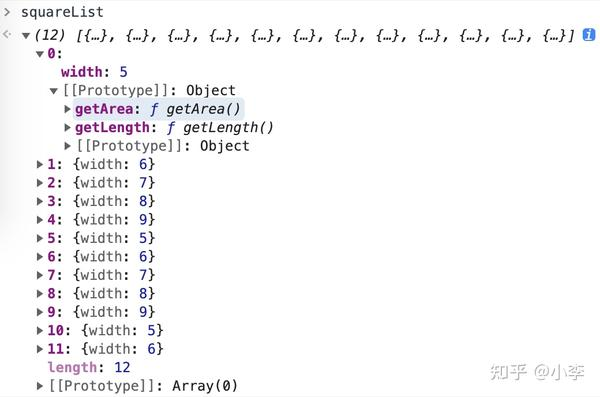let squareList=[]
let widthList=[5,6,7,8,9,5,6,7,8,9,5,6]
function createSquare(width){//此函数叫构造函数,构造函数就是可以构造出对象的函数
let obj=Object.create(squarePrototype)//以squarePrototype为原型创建空对象
obj.width=width
return obj
}
let squarePrototype={
getArea(){
return this.width * this.width
},
getLength(){
return this.width * 4
}
}
for(let i=0;i<12;i++){
squareList[i]=createSquare(widthList[i])
//封装，把细节写到一个函数里，别人只需要调用函数并且传个宽度。
}


squarePrototype原型和createSquare函数还是分散的,能不能组合在一起?

let squareList = []
let widthList = [5,6,5,6,5,6,5,6,5,6,5,6]

//声明createSquare函数，它可以创建square对象
function createSquare(width){
let obj = Object.create(createSquare.squarePrototype) // 先使用后定义？NO
这段代码在调用createSquare才会执行
obj.width = width
return obj
}
createSquare.squarePrototype = { //把原型放到函数上，结合够紧密了吗？
getArea(){
return this.width * this.width
},
getLength(){
return this.width * 4
},
constructor: createSquare //方便通过原型找到构造函数(又把构造函数放到原型上)
}
for(let i = 0; i<12; i++){
squareList[i] = createSquare(widthList[i])//这里才调用createSquare
console.log(squareList[i].constructor)
// constructor 可以知道谁构造了这个对象：你妈是谁？
}


new操作符，让我们感受js之父的爱

let squareList = []
let widthList = [5,6,5,6,5,6,5,6,5,6,5,6]
function Square(width){
this.width = width
}
Square.prototype.getArea = function(){ //设置共用属性(getArea)
return this.width * this.width
}
Square.prototype.getLength = function(){
return this.width * 4
}
for(let i = 0; i<12; i++){
squareList[i] = new Square(widthList[i])
console.log(squareList[i].constructor)
}
// 多美，几乎没有一句多余的废话
// 每个函数都有 prototype 属性，这是 JS 之父故意的
// 每个 prototype 都有 constructor 属性，也是故意的


js之父为了让new可以运行提前规定:

function f1(){}

console.dir(f1)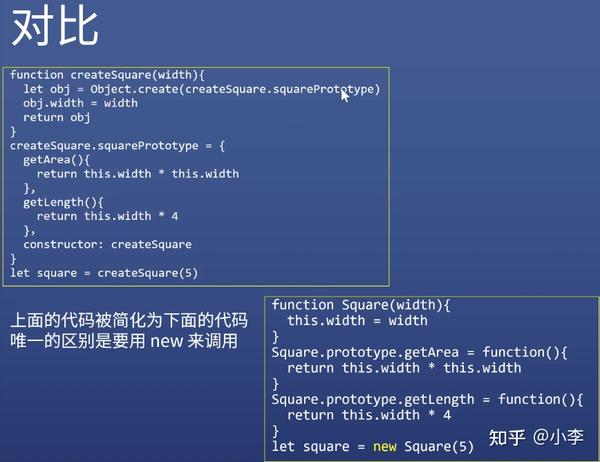1.之前要自己创建对象并把对象的原型指向那个拥有getArea,getLength的原型。

2.用this代表新的对象
3.return也不用写，new会帮我们做
4.之前会创建个对象，里面有共有属性。

1.new X()自动做了四件事情

–这就是JS之父的爱
2.构造函数X
X函数本身负责给对象本身添加属性
X.prototype对象负责保存对象的共用属性

function Dog(name){
this.name=name
this.color='白'
this.king='萨摩耶'
}
Dog.prototype.wangwang=function(){console.log('汪汪')}
Dog.prototype.run=function(){console.log('狗在跑')}
let dog1=new Dog('小白')



this指还未创建出来的新对象dog1

## 代码规范

1.大小写

function add(x,y){return x+y}  //不是构造函数不用大写
function Dog(name){this.name=name}


let dog1=new Dog()


2.词性

(1)new 后面的函数，使用名词形式

(2)其它函数，一般使用动词开头

function createSquare(width){…}

1.怎么确定要传几个参数？

function Square(width){…}

let arr1=new Array(3)


new Array(element0,element1[,…[,elementN]])//多个参数就是里面的元素

new Array(arrayLength) //一个参数就是数组的长度

## js唯一的一个公式

1.为什么

let obj=new Object()的原型是Object.prototype

let arr=new Array()的原型是Array.prototype

let square=new Square()的原型是Square.prototype

let fn=new Function()的原型是Function.prototype

2.因为new操作故意这么做的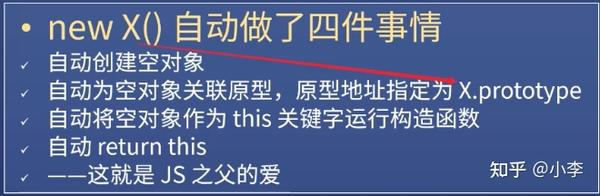new后面是什么，原型就是 对应的.prototype

let x={}

1.x的原型是什么？x.__proto__的值是什么？上面两个问题是等价的吗？

let x=new Object{}

x.proto=Object.prototype

x.__proto__存的地址才是其原型

2.用内存图画出x的所有属性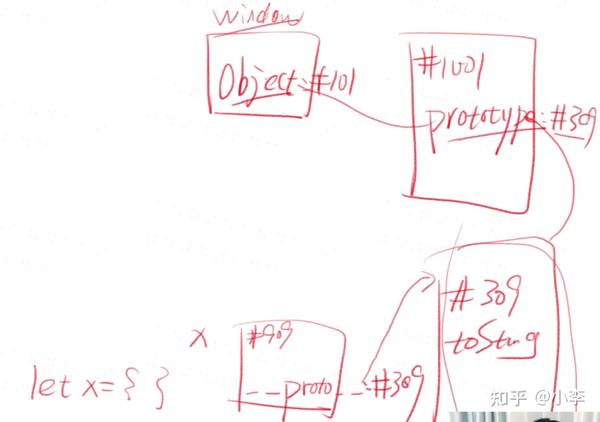let square=new Square(5)

1.square的原型是什么？square.__proto__的值是什么？

square.proto=Square.prototype

2.用内存图画出square的所有属性,略

1.Object.prototype是哪个函数构造出来的？

2.Object.prototype的原型是？

3.Object.prototype.proto?

null

4.用内存图画出上述内容,略

window.Function是一个函数对象，那么这个「函数对象」的构造函数是Function;

window.Object是一个函数对象，那么这个「函数对象」的构造函数是Function;

window.Function 是一个函数对象，那么这个「函数对象」的__proto__是(对象. proto===其构造函数.prototype）

Square最终版(存疑),还可以进一步优化，以后再说。

### 对象需要分类

function Circle(radius){
}
Circle.prototype.getArea=function(){
}
Circle.prototype.getLength=function(){
return this.radius * 2 * Math.PI
}
let c1=new Circle(10)//声明一个新的圆



function Rect(width,height){
this.width = width
this.height=height
}
Rect.prototype.getArea=function(){
return this.width * this.height
}
Rect.prototype.getLength=function(){
return (this.width+this.height)*2
}

let r1=new Rect(4,5) //声明一个新的长方形



Array/Function也是不同的分类

## object是最没有特色的类，现在来看下有特色的类

### 第一种.数组对象

1.定义一个数组

let arr=[1,2,3] //缩写

let arr=new Array(1,2,3) //元素为1，2，3

let arr=new Array(3) //长度为3

2.数组对象的自身属性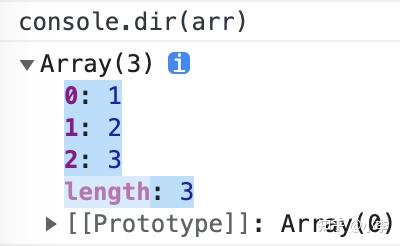3.数组对象的共用属性

### 第二种.函数对象

1.定义一个函数

function fn(x,y){return x+y}

let fn2=function fn(x,y){return x+y}

let fn=(x,y)=>x+y

let fn=new Function(‘x’,‘y’,‘return x+y’) //重要

2.函数对象自身属性

’name’/’length’

let fn = new Function('x', 'y', 'return x+y')
console.dir(fn)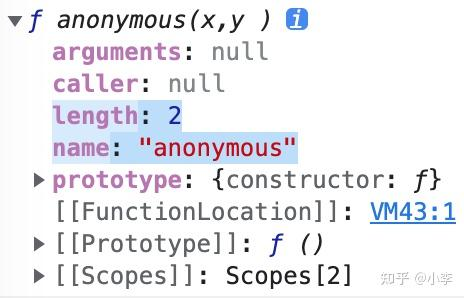3.函数对象共用属性

‘call’/‘bind’/‘apply’

### JS终极一问

1.window是谁构造的？

Window(大写)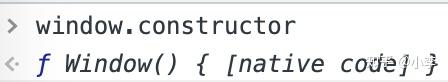2.window.Object是谁构造的？

window.Function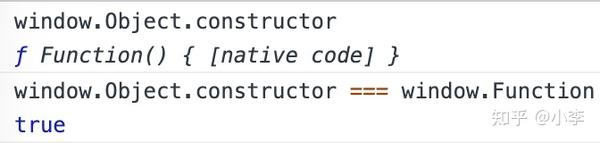3.window.Function是谁构造的？

window.Function

window.Function.constructor === window.Function

### es6新语法class

prototype语法

function Square(width){
this.width = width
}
Square.prototype.getArea = function(){ //设置共用属性(getArea)
return this.width * this.width
}
Square.prototype.getLength = function(){
return this.width * 4
}


class语法

class Square{
constructor(width){
this.width=width
}
}
getArea(){
return this.width * this.width
}
getLength(){
return this.width * 4
}


class语法引入了更多概念

class Square{
static x = 1 //第1个概念.意思是：属性(x)是Square的，Square.x即可使用x
width = 0 //第2个概念.初始化this.width(宽度默认为0)
constructor(width){
this.width=width
}
}
getArea(){
return this.width * this.width
}
getLength(){
return this.width * 4
}
get area2(){ //第3个概念.只读属性，可以将getArea()写成get area2()。区别是：调用area2时不需要加括号()
return this.width * this.width
}


class Circle{
}
getArea(){
}
getLength(){
return this.radius * 2 * Math.PI
}
}
let circle = new Circle(5)
circle.getArea()
circle.getLength()

circle.getLength()


1.这么多新语法怎么学

1’ MDN class文档全部看完，并记下来

2’ 用到再学,实践中学，记得更牢

ES 6的所有新语法 关于类和对象的新语法有对象初始化解构赋值

### class 中2种函数写法的区别

class Person{
sayHi(name){}
//等价于
sayHi: function(name){}
//注意，一般我们不在这个语法里使用箭头函数
}

//等价于

function Person(){}
Person.prototype.sayHi = function(name){}


class Person{
sayHi = (name)=>{} // 注意，一般我们不在这个语法里使用普通函数，多用箭头函数
}

//等价于

function Person(){
this.sayHi = (name)=>{}
}


class就是声明一个类，类就是用来创建对象的，只需要按照这个语法写就可以了。

Previous JS对象